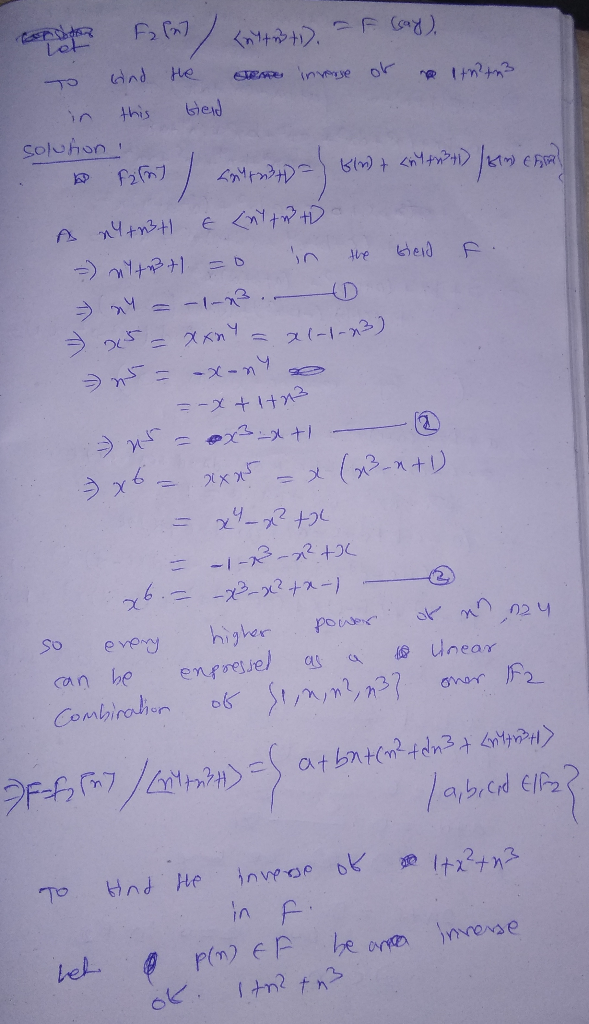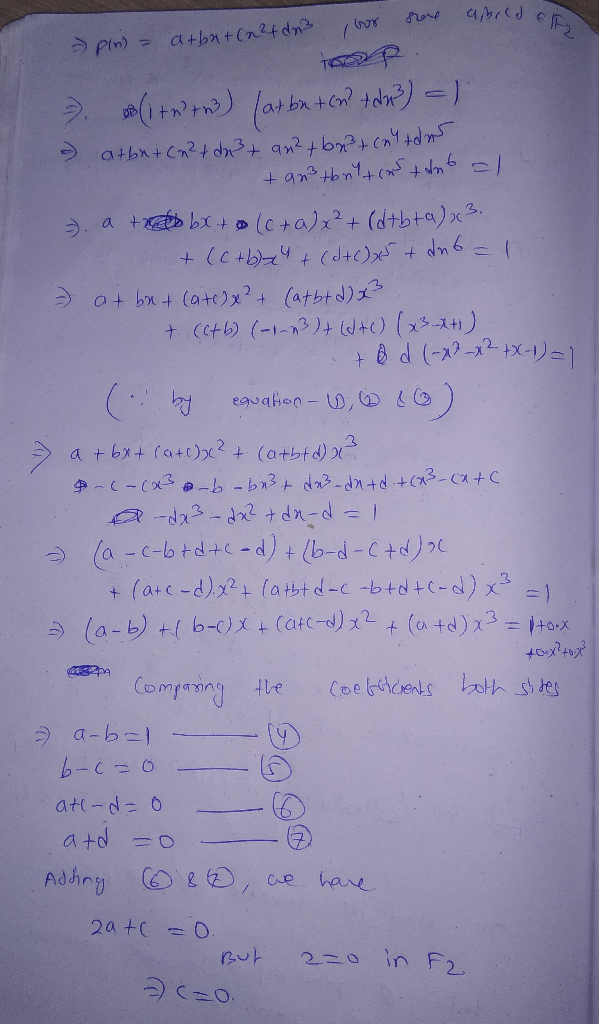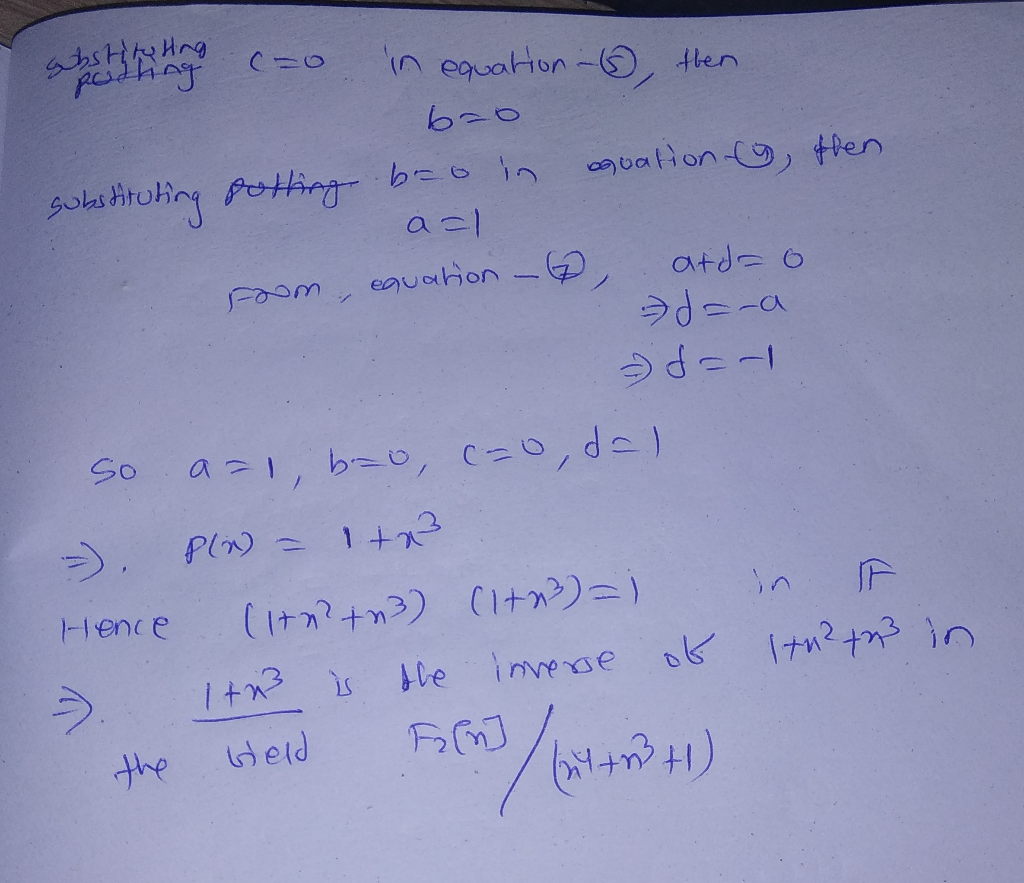# Find the inverse of the element x^2 + x^3 + 1 in the field F2[x] / (x^4 + x^3 +1)

Find the inverse of the element x^2 + x^3 + 1 in the field F2[x] / (x^4 + x^3 +1)##### Add Answer of: Find the inverse of the element x^2 + x^3 + 1 in the field F2[x] / (x^4 + x^3 +1)
More Homework Help Questions Additional questions in this topic.